Main Content

# Using MPC Controller Block Inside Function-Call and Triggered Subsystems

This example shows how to configure and simulate MPC Controller blocks placed inside Function-Call and Triggered subsystems.

### Define Plant Model and MPC Controller

Define a SISO plant.

```plant = ss(tf([3 1],[1 0.6 1])); ```

Define the MPC controller for the plant.

```Ts = 0.1; %Sampling time p = 10; %Prediction horizon m = 2; %Control horizon Weights = struct('MV',0,'MVRate',0.01,'OV',1); % Weights MV = struct('Min',-Inf,'Max',Inf,'RateMin',-100,'RateMax',100); % Input constraints OV = struct('Min',-2,'Max',2); % Output constraints mpcobj = mpc(plant,Ts,p,m,Weights,MV,OV); ```

### Configure and Simulate MPC Controller Block Inside Function-Call Subsystem

To run this example, Simulink® is required.

```if ~mpcchecktoolboxinstalled('simulink') disp('Simulink(R) is required to run this example.') return end ```

Function-Call subsystem is invoked directly by another block during simulation. To ensure that the MPC controller works properly inside a Function-Call subsystem, you must configure the MPC Controller block to use inherited sample time and invoke the Function-Call subsystem periodically with the same sample time defined in the MPC controller object.

Open the model.

```mdl1 = 'mpc_rtwdemo_functioncall'; open_system(mdl1) ```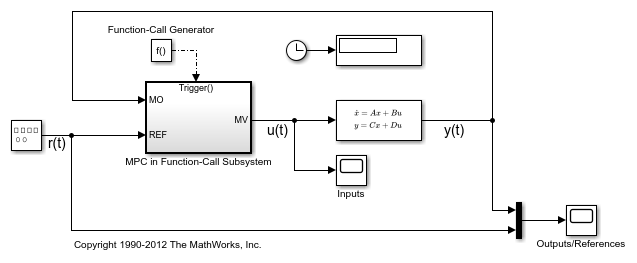The MPC Controller block is inside the MPC in Triggered Subsystem block.

```open_system([mdl1 '/MPC in Function-Call Subsystem']) ```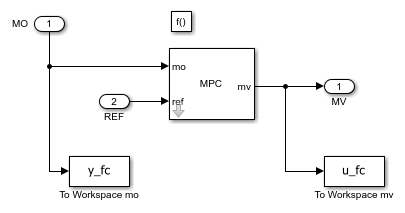Configure the controller to use an inherited sample time. To do so, select the Inherit sample time property of the MPC Controller block.

Invoke the Function-Call subsystem periodically with the correct sample time.

For this example, since the controller has a sample time of 0.1 seconds, configure the trigger block inside the Function-Call subsystem to use the same sample time.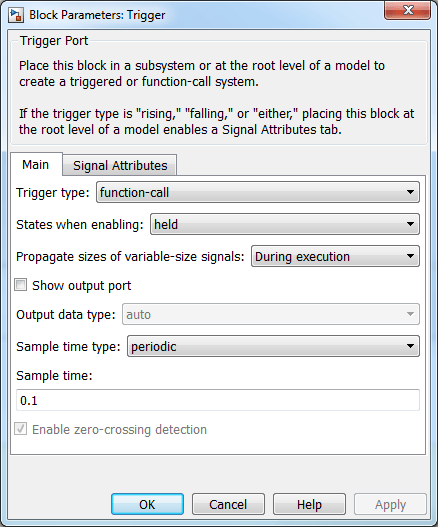For this example, use the Function-Call Generator block to execute the Function-Call subsystem at the sample rate as 0.1 seconds.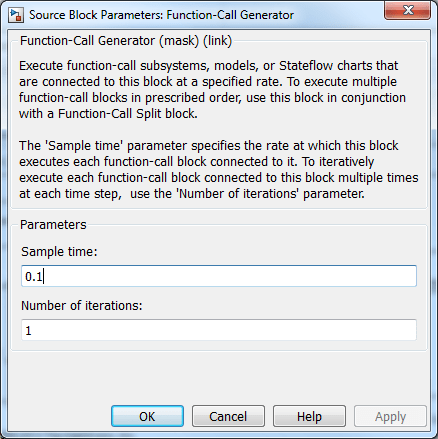Simulate the model.

```close_system([mdl1 '/MPC in Function-Call Subsystem/MPC Controller']) open_system([mdl1 '/Inputs']) open_system([mdl1 '/Outputs//References']) sim(mdl1) ```
```-->Converting model to discrete time. -->Assuming output disturbance added to measured output channel #1 is integrated white noise. -->The "Model.Noise" property of the "mpc" object is empty. Assuming white noise on each measured output channel. ```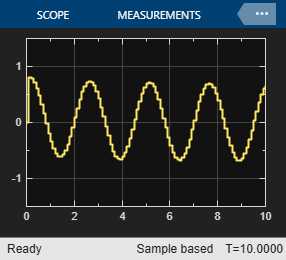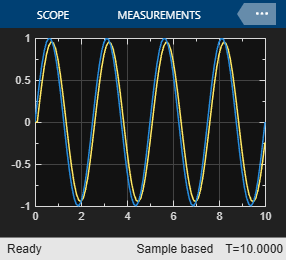The controller effort and the plant output are saved into base workspace as variables `u_fc` and `y_fc`, respectively.

Close the Simulink model.

```bdclose(mdl1) ```

### Configure and Simulate MPC Controller Block Inside Triggered Subsystem

Triggered subsystem executes each time a trigger event occurs. To ensure that the MPC controller works properly inside a triggered subsystem, you must configure the MPC Controller block to use inherited sample time and invoke the Triggered subsystem periodically with the same sample time defined in the MPC controller object.

Open the model.

```mdl2 = 'mpc_rtwdemo_triggered'; open_system(mdl2) ```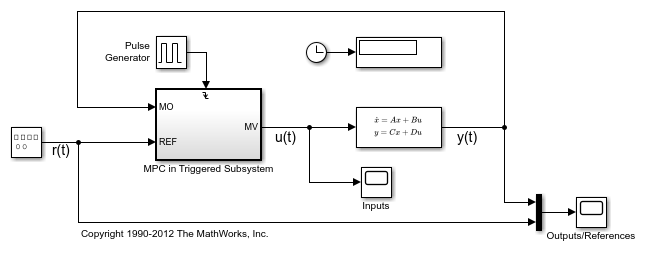The MPC Controller block is in the MPC in Triggered Subsystem block.

```open_system([mdl2 '/MPC in Triggered Subsystem']); ```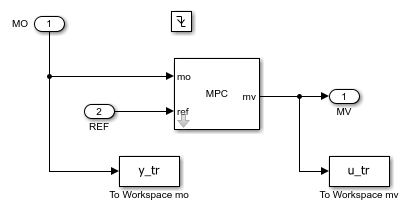Configure the MPC block to use an inherited sample time, as you did for the function-call subsystem model.

Execute the Triggered subsystem periodically with the correct sample time.

For this example, configure the Trigger block inside the triggered subsystem to use a `falling` trigger type.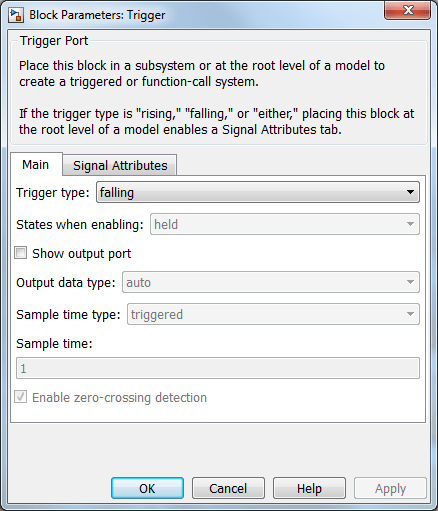For this example, use the Pulse Generator block to provide a periodic triggering signal at the sample rate as 0.1 seconds.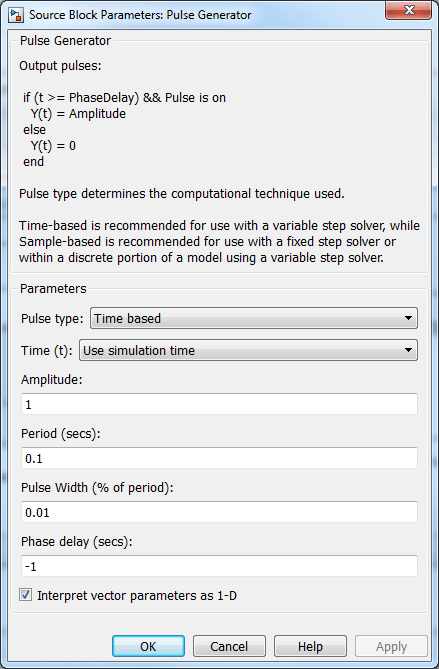Simulate the model.

```close_system([mdl2 '/MPC in Triggered Subsystem/MPC Controller']) open_system([mdl2 '/Inputs']) open_system([mdl2 '/Outputs//References']) sim(mdl2) ```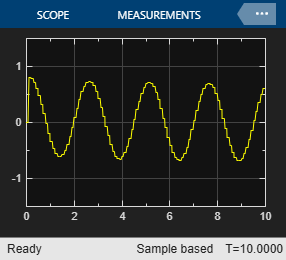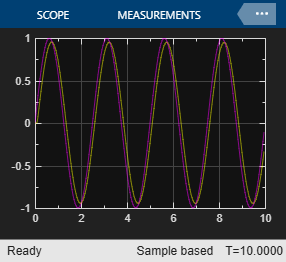The controller effort and the plant output are saved into base workspace as variables `u_tr` and `y_tr`, respectively.

Close the Simulink model.

```bdclose(mdl2) ```

### Compare Responses

Compare the simulation results from the Function-Call subsystem and the Triggered subsystem with the result generated by an MPC Controller block that is not placed inside a subsystem and does not inherit sample time.

```mdl = 'mpc_rtwdemo'; open_system(mdl) sim(mdl) ```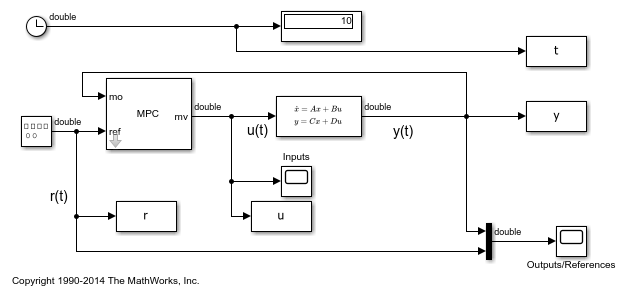Compare the responses of the manipulated variable.

```figure plot(t,u,'b-',t,u_fc,'ro',t(1:end-1),u_tr,'k.') title('Manipulated Variable') legend('No Subsystem','Function-Call','Triggered') ```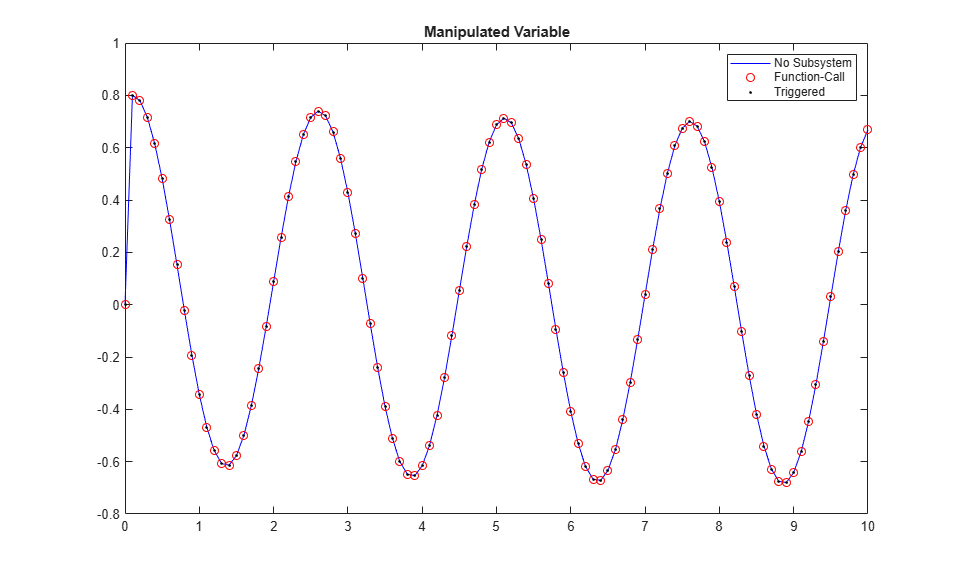Compare the responses the plant output.

```figure plot(t,y,'b-',t,y_fc,'ro',t(1:end-1),y_tr,'k.') title('Plant Output') legend('No Subsystem','Function-Call','Triggered') ```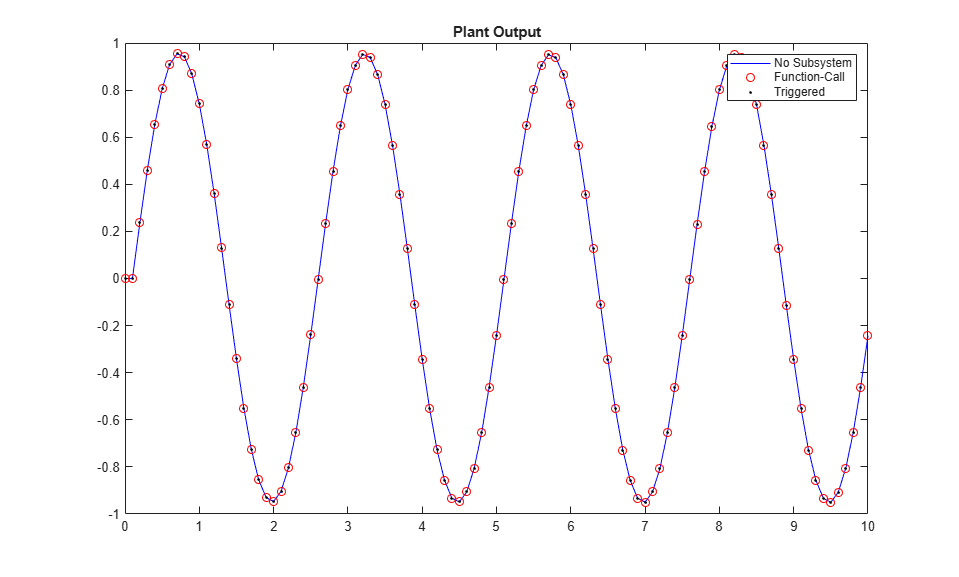The results of all three models are numerically equal.

Close the Simulink model.

```bdclose(mdl) ```

## See Also

(Simulink) | (Simulink)

## Support

#### Implementing an Adaptive Cruise Controller with Simulink

Download technical paper